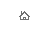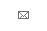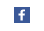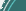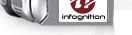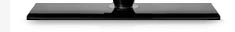blog tags: directshow (8) programming (11) super_resolution (6) video (2) video_enhancer (12) all (40) About: I'm Dmitry Popov, lead developer and director of Infognition. Known in the interwebs as Dee Mon since 1997. You could see me as thedeemon on reddit or LiveJournal.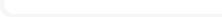Articles Technology Blog News Company
Blog
 Recursive algebraic types in D September 15, 2014 One of the things D programming language seemingly lacks is support for algebraic data types and pattern matching on them. This is a very convenient kind of types which most functional languages have built-in (as well as modern imperative ones like Rust or Haxe). There were some attempts at making algebraic types on the library level in D (such as Algebraic template in std.variant module of D's stdlib) however they totally failed their name: they don't support recursion and hence are not algrabraic at all, they are sum types at best. What follows is a brief explanation of the topic and a proof of concept implementation of recursive algebraic types in D. So what the heck are algebraic types after all? They must have something to do with algebra, right? In school we spent a lot of time in algebra classes working with functions, equations and searching for their roots but nobody told us what an algebra, as a mathematical object, actually is. An algebra is often defined as some set together with a collection of operations on elements of this set. We programmers and computer science enthusiasts work with types instead of sets (type is a more general thing than a set btw). So, in school we dealt with some particular examples of algebras. For example, we used set R (the set of all real numbers) and operations like x+y, x-y, x*y, xy, -x (negation, let's write it as ~x) etc. We can write their types as: ```+: (R, R) -> R -: (R, R) -> R *: (R, R) -> R ~: R -> R power: (R, R) -> R ... ``` Each of these operations takes some fixed number of elements of type R and outputs one element of R. Constants can be thought of as operations taking zero R arguments and returning one, e.g. ```42: () -> R ``` We can also replace R with Q (the set of all rational numbers) and have most of these operations work on Q. That would be another algebra. It's crucial that operations take arguments from the same set (type) as results they produce. That is algebraic. So, encoded in a programming language, a real algebraic type must support recursion. Otherwise it's just a sum type, discriminated union, not very useful for encoding expressions, syntax trees, other kinds of trees or even lists. The list of operations above is already looking very much like a generalized algebraic data type definition in functional languages like Haskell, OCaml, Agda or Idris. Here is how it's written in OCaml: ```type r = | Add : r * r -> r | Sub : r * r -> r | Mul : r * r -> r | Neg : r -> r | C42 : () -> r ``` In Haskell it's essentially the same, modulo currying: ```data R where Add :: R -> R -> R Sub :: R -> R -> R Mul :: R -> R -> R Neg :: R -> R C42 :: () -> R ``` Since all these operations provide result of the same simple type R, we can use the simpler syntax: ```data R = Add R R | Sub R R | Mul R R | Neg R | C42 ``` Note that here we only define names and form (types) of operations, not their real content. If we change R to Q we get essentially the same type, only renamed. Unlike in math, where when switching from set R to set Q we also changed the meaning (implementations if you wish) of functions: from adding / subtracting / etc. real numbers to adding / subtracting / etc. rational ones. To emphasize the fact that ADT only describes the names and shapes of algebra operations, we can replace occurences of R in operands by a type variable: ```data F a = Add a a | Sub a a | Mul a a | Neg a | C42 ``` In order to encode a particular algebra with such operations we need, besides this definition, a type `T` of values (the algebra carrier) and an evaluation function ```eval : F T -> T ``` This function will do a case analysis ("which operation is encoded?") and perform the operation, because it knows well how to add, multiply, negate and so on values of this type `T`. Now let's recall some category theory. A category consists of a collection of objects and a collection of arrows (also called morphisms) between those objects, with some required properties regarding their compositions and identity morphisms. We're interested in a category where objects are types and arrows are functions so that each function `f` with argument of type `A` and return value of type `B` is an arrow from `A` to `B`, i.e. `f : A -> B`. A functor is a mapping from one category to another, it maps objects of one category to objects of another and the arrows are mapped correspondingly, so that all the compositions remain. Functor mapping a category to itself is called an endofunctor. The parameterized type `F` above is such an endofunctor: any type `X` it maps to type `F X`, and any function `g : X -> Y` can be trivially mapped to a fuction ```fmap g : F X -> F Y ``` (Haskell compiler can derive the implementation of fmap here) Now let's look at definition of F-Algebra in category theory. It consists of three parts: an endofunctor `F`, object `T` and a morphism from `F(T)` to `T`. These are exactly the three things mentioned above that we need to encode some particular algebra. No wonder. Ok, one more thing before we get to code in D. Recursion. Describing a functor like `F` above is simple but we want the type to be recursive: operations like Add should have operands of same type as their result. Their operands' type is described by type parameter `a`, their result is `F a`, and they somehow must be equal. I.e. we need to find a type `X` to be used as type parameter to `F` such that ```F X = X ``` Looks like we need the fixed point of `F`. Turns out we can define it quite easily. In Haskell it looks like this: ```newtype Fix f = Fx (f (Fix f)) ``` together with unwrapping operation ```unFix :: Fix f -> f (Fix f) unFix (Fx x) = x ``` and in D it looks like this: ```struct Fix(alias F) { F!(Fix!F) unFix; } ``` `F`, being a functor, shall be some struct or class template, so we pass it by `alias`. (Higher kinded types? we can has them!) For any endofunctor `f`, `Fix f` is a type, an object in our category. This functor, this object and the arrow `Fx: f (Fix f) -> Fix f` together form an F-algebra. Category theory says this F-algebra is special: it's the initial object in the category of F-algebras for this functor, which means for any other algebra for this functor (say, object `T` and its evaluator arrow `alg: F T -> T`) there is a unique morphism `g` from initial algebra `Fix f` to `T`, such that this diagram commutes: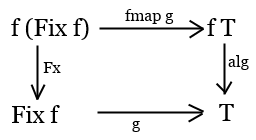(commutes - means the two paths from `f (Fix f)` to `T` are equal) Knowing that `unFix` is the inverse of `Fx` we can actually define `g` via `unFix, fmap g` and `alg`.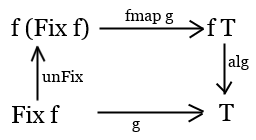This unique morphism from initial object is called catamorhism, so we'll call it `cata` with `alg` as its parameter. In Haskell it looks like this: ```cata :: Functor f => (f a -> a) -> Fix f -> a cata alg = alg . fmap (cata alg) . unFix ``` And in D it looks like this: ```T cata(alias F, T)(T function(F!T) alg, Fix!F e) { return alg( e.unFix.fmap( (Fix!F x) => cata!(F,T)(alg, x) ) ); } ``` Here we employ the fact that `F` is a functor and hence has `fmap` function defined. Having the catamorphism allows us to easily define different evaluation functions for our recursive algebraic type from their non-recursive versions defined for particular algebras. I think it is time to look at the code with some examples to understand how that works. For simplicity let's take a very tiny algebra: it will contain integer numbers and one addition operation. Something like this: ```data Exp = Add Exp Exp | Const Int ``` Algebraic data types usually consist of a sum of products. Product types we had in C-like languages for ages: structs and classes. Sum types D doesn't have built-in, but we can make them with templates. The simplest sum type would be a sum of two types, A and B, known in Haskell as `Either a b`. Its implementation as a discriminated union is very straightforward, with one twist: as shown above, we really need a functor, a template in D, and the summands must have a type parameter too, so they will be also templates. I'll also add a `match` function to do familiar by functional languages pattern matching on the values. Here's how our Either will look in D: ```// A and B are class(T) or struct(T) implementing fmap template Either(alias A, alias B) { class EitherImpl(T) { enum Tag { kA, kB } Tag tag; union { A!T vA; B!T vB; } this(A!T a) { tag = Tag.kA; vA = a; } this(B!T b) { tag = Tag.kB; vB = b; } U match(U)(U delegate(A!T) fa, U delegate(B!T) fb) { final switch(tag) { case Tag.kA: return fa(vA); case Tag.kB: return fb(vB); } } EitherImpl!U fmap(U)(U delegate(T) f) { return match(a => new EitherImpl!U( a.fmap(f) ), b => new EitherImpl!U( b.fmap(f) )); } } alias Either = EitherImpl; } ``` The functor for our little algebra of expressions will be defined as a sum ```alias Exp = Either!(Add, Const); ``` where the two summands are ```struct Const(T) { int x; mixin Functor!(Const, T); } class Add(T) { T l, r; this(T a, T b) { l = a; r = b; } this() {} mixin Functor!(Add, T, "l", "r"); } ``` The `mixin Functor` line plays the same role as `(deriving Functor)` in Haskell. It's a piece of metaprogramming in D to define `fmap` methods: ```mixin template Functor(alias F,T, Vars...) { F!U fmap(U)(U delegate(T) f) { static if (is(typeof(this)==class)) auto r = new F!U; else auto r = F!U(); foreach(m; __traits(allMembers, typeof(r))) static if (m != "Monitor" && m != "fmap") { static if (IndexOf!(m, Vars) >= 0) __traits(getMember, r, m) = f(__traits(getMember, this, m)); else static if (!isSomeFunction!(typeof(__traits(getMember, r, m))) && isAssignable!(typeof(__traits(getMember, r, m)))) __traits(getMember, r, m) = __traits(getMember, this, m); } return r; } } ``` It's ugly but only needs to be defined once. For a class or struct template `F!T` it defines a method `fmap` that can take any function `f` of type `T -> U` and make a similar object or struct of type `F!U` where given fields will be mapped by function `f` while others will be simply copied. After having a functor `Exp` defined using `Either` we need to turn it into a recursive type by using its fix point: ```alias FixX = Fix!Exp; alias Exprec = Exp!FixX; ``` A small helper for creating its values would be handy: ```auto mk(alias T, Xs...)(Xs xs) { static if (is(T!FixX==class)) auto x = new T!FixX(xs); else auto x = T!FixX(xs); return FixX(new Exprec(x)); } ``` Now we can create some expressions in our little algebra: ``` auto n1 = mk!Const(5); auto n2 = mk!Const(7); auto e1 = mk!Add(n1, n2); auto e2 = mk!Add(mk!Const(30), e1); // e2 is (30 + (5 + 7)) ``` What do we do with them? We'd like to calculate expressions to some int results and we want to output expressions as strings. Each operation can be described as an algebra with int or string type as its carrier and corresponding evaluation function from `Exp!int` or `Exp!string` to int or string. Here they are: ```//alg : f a -> a, for some concrete a int eval(Exp!int e) { return e.match(a => a.l + a.r, i => i.x); } string show(Exp!string e) { return e.match(a => "(" ~ a.l ~ " + " ~ a.r ~ ")", i => i.x.text); } ``` They use pattern matching by passing to lambdas to `match`: one that tells what to do with Add case and one that tells how to process the Const case. These are not recursive and describe just one step of computations. Now we can use our catamorphism to apply them recursively and turn such function of type `f a -> a` into function of type `Fix f -> a` which will give us desired results: ``` cata(&show, e2).writeln; // => (30 + (5 + 7)) cata(&eval, e2).writeln; // => 42 ``` Voila! The full source code, compilable and runnable, can be found here on dpaste.Comments (16) tags: programming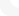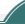Copyright © 2001-2009 Dee Mon, 2009-2020 Infognition Co. Ltd.